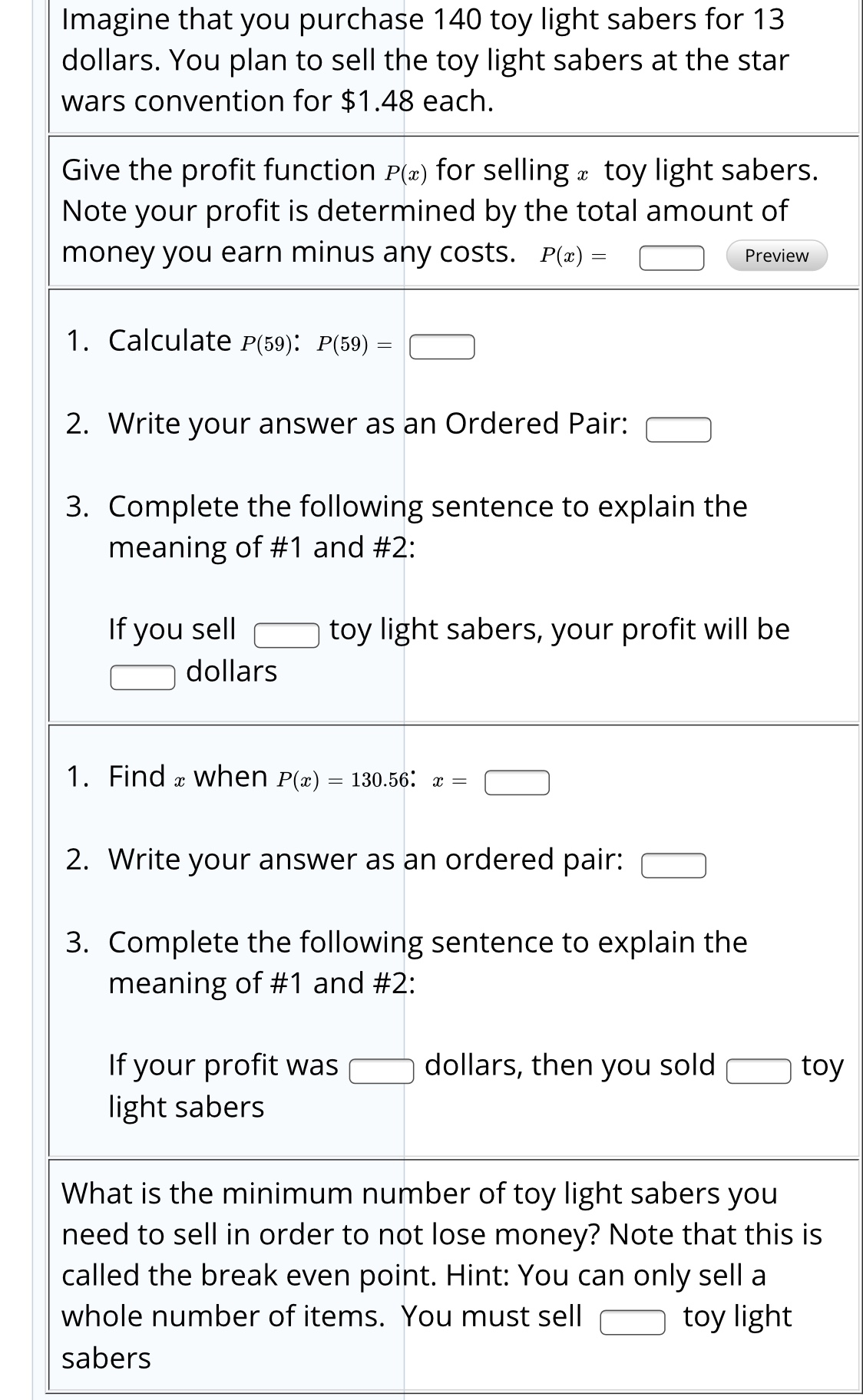# Imagine that you purchase 140 toy light sabers for 13dollars. You plan to sell the toy light sabers at the starwars convention for \$1.48 each.Give the profit function P(a) for selling a toy light sabers.Note your profit is determined by the total amount ofmoney you earn minus any costs. P(x) =Preview1. Calculate P(59): P(59)2. Write your answer as an Ordered Pair:3. Complete the following sentence to explain themeaning of #1 and #2:toy light sabers, your profit will beIf you selldollars1. Find æ when P(x)130.56: x =2. Write your answer as an ordered pair:3. Complete the following sentence to explain themeaning of #1 and #2:If your profit waslight sabersdollars, then you soldtoyWhat is the minimum number of toy light sabers youneed to sell in order to not lose money? Note that this iscalled the break even point. Hint: You can only sell awhole number of items. You must selltoy lightsabers

Question
19 viewshelp_outlineImage TranscriptioncloseImagine that you purchase 140 toy light sabers for 13 dollars. You plan to sell the toy light sabers at the star wars convention for \$1.48 each. Give the profit function P(a) for selling a toy light sabers. Note your profit is determined by the total amount of money you earn minus any costs. P(x) = Preview 1. Calculate P(59): P(59) 2. Write your answer as an Ordered Pair: 3. Complete the following sentence to explain the meaning of #1 and #2: toy light sabers, your profit will be If you sell dollars 1. Find æ when P(x) 130.56: x = 2. Write your answer as an ordered pair: 3. Complete the following sentence to explain the meaning of #1 and #2: If your profit was light sabers dollars, then you sold toy What is the minimum number of toy light sabers you need to sell in order to not lose money? Note that this is called the break even point. Hint: You can only sell a whole number of items. You must sell toy light sabers fullscreen
check_circle

Step 1

Hello. Since your question has multiple sub-parts, we will solve first three sub-parts for you. If you want remaining sub-parts to be solved, then please resubmit the whole question and specify those sub-parts you want us to solve.

Consider the calculation for (1).

Let the number of units of toy light sabers be x.

Calculate the cost price for the x units of light sabers.

Then the function for the cost price is C(x).

Calculate the selling price for the x units of light sabers.

Then the function for the revenue is R(x).

Step 2

Then, consider the formula for the profit function P(x).

Substitute the value of R(x) and C(x) in the P(x) and simplify to calculate P(x).

Calculate the value of P (59).

Step 3

Consider the calculation for (2).

The value of P (59) is 81.833.

Hence, th...

### Want to see the full answer?

See Solution

#### Want to see this answer and more?

Solutions are written by subject experts who are available 24/7. Questions are typically answered within 1 hour.*

See Solution
*Response times may vary by subject and question.
Tagged in